## 边坡稳定分析的虚功率法 1)

*中国科学院力学研究所, 北京 100190

**中国电建集团贵阳水利水电勘测设计研究院, 贵阳 550081

## A VIRTUAL POWER SLOPE STABILITY ANALYSIS METHOD 1)

Wu Mengxi,*,,2), Yang Jiaxiu**, Zhan Zhenggang**

*Institude of Mechanics, Chinese Academy of Sciences, Beijing 100190, China

University of Chinese Academy of Sciences, Beijing 100049, China

**Powerchina Guiyang Engineering Corporation Limited, Guiyang 550081, China

 基金资助: 1)国家重点研发计划.  2017YFC1501100

Received: 2019-02-15   Accepted: 2020-04-1   Online: 2020-05-18Abstract

The improved limit equilibrium method, which bases on the finite element stress field to analyze the stability of a slope, has an advantage in the analysis of the stability of a slope or a foundation with complex geological composition and strong coupling effect of seepage and effective stresses. In this paper, a virtual power method for slope stability analysis is proposed. The safety factor is calculated by using the ratio of anti-slip power to sliding power on the velocity discontinuities of the sliding mechanism by using the permissible velocity field for maneuvering of the combined rigid body sliding mechanism and the finite element stress field. The stability safety factor of a given sliding mechanism of the slope is obtained by the method of a step by step optimization strategy. Two typical sliding slope cases with weak interlayers are analyzed, including the comparison of the influence to the safety factor results of the stress fields whether static equilibrium only or also hydrostatic allowable. The safety factor in virtual power safety factor method based on linear elastic effective stress field is still a good measure to the stability of a slope even if it is not the best while the stress field is not static permissible. Slope safety factors calculated here are very close to the reference answers in the literature, the sliding mechanisms are consistent with and the safety coefficients are close to that of by the strength reduction finite element method. These show that the proposed method is reasonable. The proposed method is an alternative for the stability analysis of slopes and foundations.

Keywords： slope stability ; finite element method ; virtual power method ; upper bound theorem

Wu Mengxi, Yang Jiaxiu, Zhan Zhenggang. A VIRTUAL POWER SLOPE STABILITY ANALYSIS METHOD 1). Chinese Journal of Theoretical and Applied Mechanics[J], 2020, 52(3): 663-672 DOI:10.6052/0459-1879-20-040

## 1 边坡稳定分析的虚功率法理论

### 图1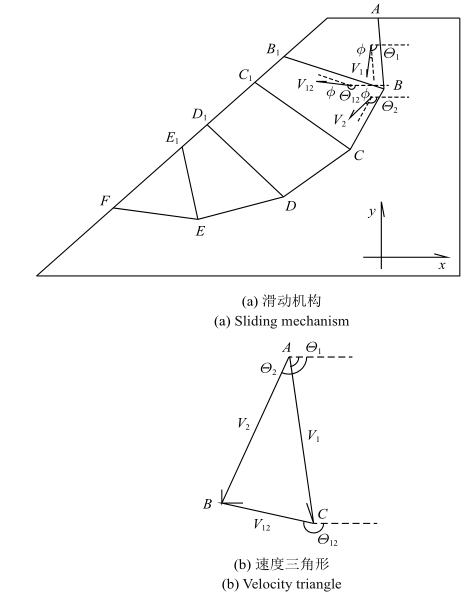Fig.1

$P=\int_\varGamma v\tau \cos \phi {d}\varGamma +\int_\varGamma v\sigma _n \sin \phi {d}\varGamma$

$\int_\varGamma v\tau \cos \phi {d}\varGamma +\int_\varGamma v\sigma _n \sin \phi {d}\varGamma = \\ \qquad\int_\varGamma v(\tau _{f} /F)\cos \phi {d}\varGamma +\int_\varGamma v\sigma _n \sin \phi {d}\varGamma$

$\tau _{f} =c+\sigma _n \tan \varphi$

$F=\frac{\int_\varGamma v\tau _{f} \cos \phi {d}\varGamma}{\int_\varGamma v\tau \cos \phi {d}\varGamma}$

### 1.2 滑动面上剪切力与抗剪强度的计算

$\sigma _{ij} =\sigma _{ijJ}^{node} N_J$

$T_i =\sigma _{ij} n_j$

$\sigma _n =T_i n_i$
$\tau =T_i l_i$

### 1.3 边坡组合滑动机制与速度场

$\frac{V_1 }{\sin \angle B}=\frac{V_2 }{\sin \angle C}=\frac{V_{12} }{\sin \angle A}$

## 2 边坡稳定安全系数计算检验

1987年Donald 教授和Giam博士主持了澳大利亚计算机协会(ACADS)对澳大利亚所使用的边坡稳定分析程序进行了调查研究. 共设计了5个考题总计10个小题, 向120个单位发出测试邀请, 28个单位发回了计算答案. Donald教授也使用自编的GWEDWEM和EMU程序分析了考题, 并请以色列Baker教授用SSA程序、中国的陈祖煜教授用STAB程序和加拿大的Fredlund教授提供裁判答案. 最终综合各单位提供的答案、裁判答案和Donald教授的计算结果给出了推荐答案. 其中的第3题(EX3)的第2小题和第4题(EX4)是测试非圆弧滑动的, 用来检验本文理论方法的合理性.

### 2.1 算例介绍

EX3考题的边坡几何剖面和有限元网格见图2. 材料参数见表1. 地下水位位于软弱夹层(2#土)底部, 地下水位以上孔压为零. 第1问为圆弧或折线滑动面的最小安全系数(EX3-1), 第二问为计算指定的折线滑动面ABCD(控制点坐标如表2)的安全系数(EX3-2).

### 图2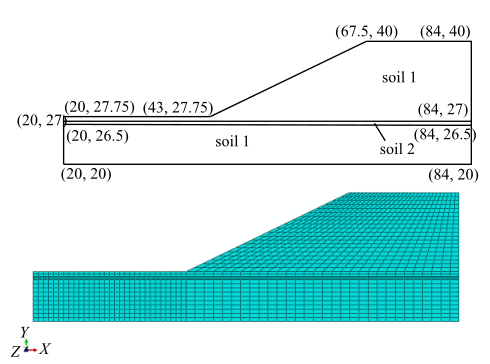Fig.2   Slope geometry, the specified polygonal sliding surface and the finite element mesh in EX3

Table 1  Material parameters in EX3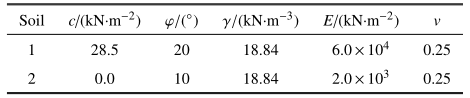Table 2  Control points of the specified polyline sliding surface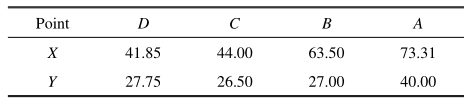### 图3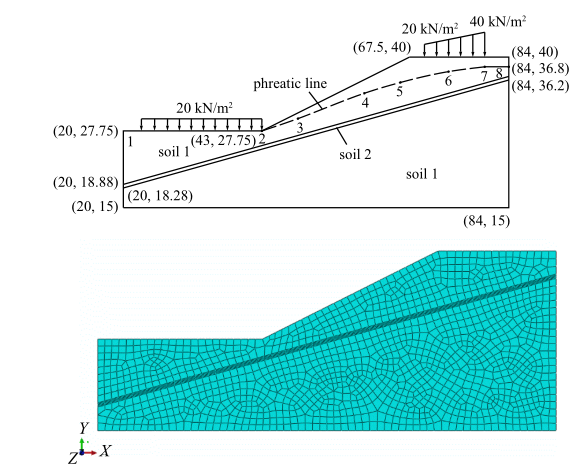Fig.3   Slope profile, load condition and the finite element mesh in EX4

Table 3  Material parameters in EX4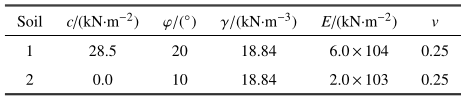Table 4  Phreatic line position in EX4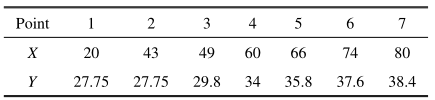### 2.2 应力场计算条件

EX3的渗流场比较简单, 是浸润线位于弱夹层底部, 该部位以下孔隙水压力为静水压力, 其上为0孔隙水压力. EX4原题只提供了浸润线, 而有限元应力计算必须要有节点孔隙水压力. 有限元渗流计算中夹层的渗透系数取1#土渗透系数的50倍, 右边界取水面高程38.4 m的等水头边界条件, 左边界及台地地表取27.75 m水头边界, 斜坡取透水边界条件, 所得边坡渗流场浸润线与等孔压线如图4所示, 图中可见浸润线位置与原题所给有一定差异.

### 图4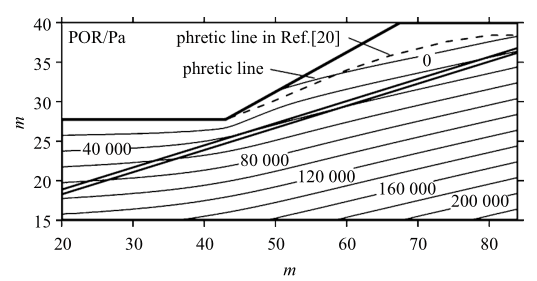Fig.4   The phreatic line and pressure contour in EX4

### 2.3 安全系数结果与文献结果的比较

Table 5  Comparison of safety coefficient results of examples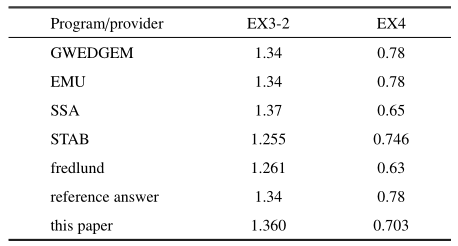### 图5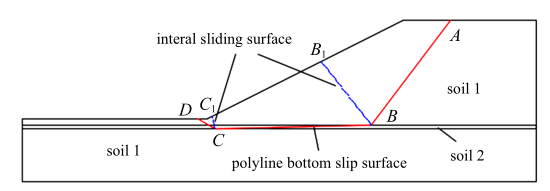Fig.5   Position of the sliding surface in EX3

Table 6  Coordinates of initial and optimum (in parentheses) points on the sliding surface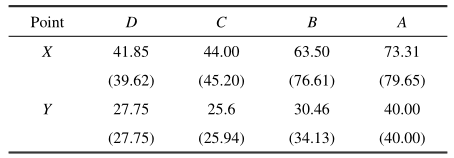### 图6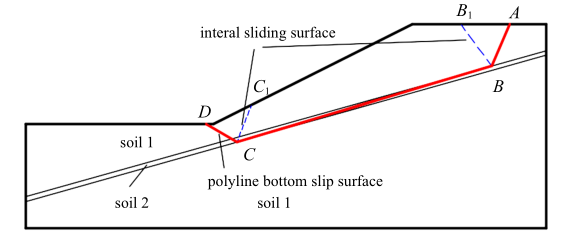Fig.6   The optical sliding surface position in EX4

### 图7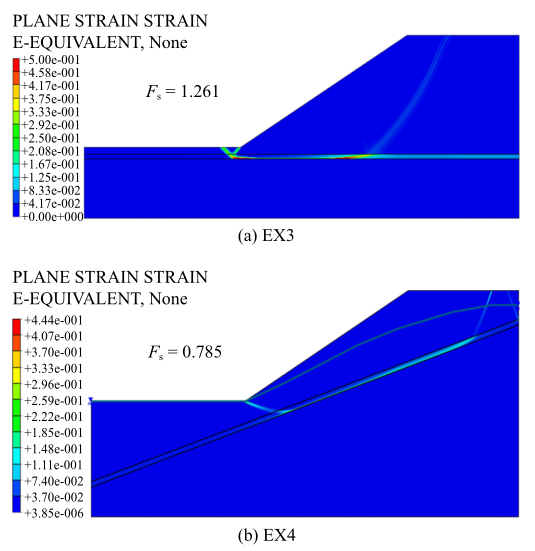Fig.7   The plastic zone and safety factor calculated by strength reduction method

### 2.5 应力场结果差异对安全系数的影响

Table 7  Stress condition and azimuth angle of optimal subslip surfaces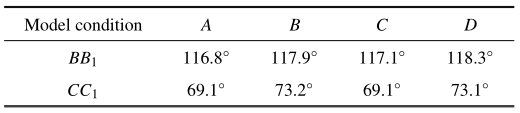Table 8  Stress condition and safety factor of polyline sliding surface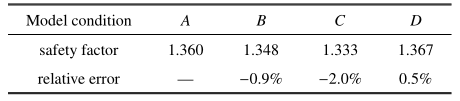Table 9  Stress condition and safety factor of polyline sliding surface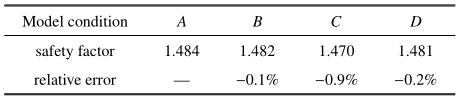## 3 结论和展望

(1) 虚功率法用边坡组合刚体滑动机动许可的速度间断面上抗滑力功率与滑动力功率之比计算安全系数, 解决了改进的极限平衡法中对于复杂滑动面安全系数计算公式物理含义不清晰的问题;

(2) 采用分步优化方法对组合滑动机制的速度间断面的位置优化, 能较容易准确确定最危险滑动机制;

(3) 虚功率法的应力计算独立于安全系数的计算与优化过程, 因而能考虑复杂的地质和渗流与应力耦合作用情况;

(4)采用静力许可的应力场, 安全系数结果更接近实际, 静力平衡的应力场, 其稳定安全性计算结果, 也是边坡稳定性的一个不错的度量.

## 致谢:

/

 〈〉